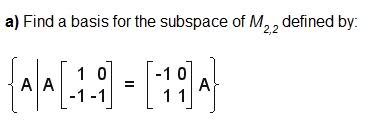# Find a basis for the subspace of M2,2

Xingconan

## Homework Statement## The Attempt at a Solution

I don't really know how to do this, so I hope someone can give some hints or briefly tell me what I should do.

## Answers and Replies

$$\left[\begin{array}{cc}a_{11} & a_{12} \\ a_{21} & a_{22}\end{array}\right]\left[\begin{array}{cc}1 & 0 \\ -1 & -1\end{array}\right]= \left[\begin{array}{cc}-1 & 0 \\ 1 & 1 \end{array}\right]$$